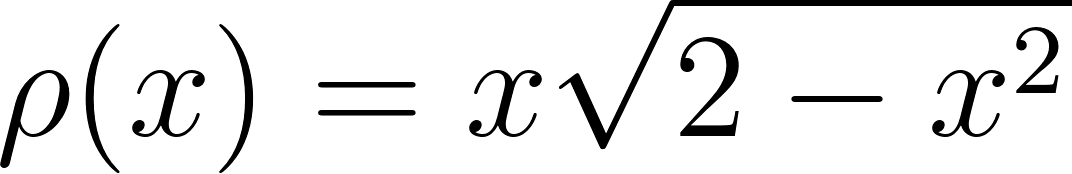×
Get Full Access to Calculus: Early Transcendentals - 1 Edition - Chapter 6.6 - Problem 13e
Get Full Access to Calculus: Early Transcendentals - 1 Edition - Chapter 6.6 - Problem 13e

×

# Mass of one-dimensional objects Find the mass | Ch 6.6 - 13EISBN: 9780321570567 2

## Solution for problem 13E Chapter 6.6

Calculus: Early Transcendentals | 1st Edition

• Textbook Solutions
• 2901 Step-by-step solutions solved by professors and subject experts
• Get 24/7 help from StudySoup virtual teaching assistantsCalculus: Early Transcendentals | 1st Edition

4 5 1 354 Reviews
29
0
Problem 13E

Mass of one-dimensional objects Find the mass of the following thin bars with the given density function.for.

Step-by-Step Solution:

Step 1 of 3

In order to find the mass of the following density function,for.

Step 2 of 3

Step 3 of 3

##### ISBN: 9780321570567

This textbook survival guide was created for the textbook: Calculus: Early Transcendentals, edition: 1. Since the solution to 13E from 6.6 chapter was answered, more than 393 students have viewed the full step-by-step answer. The answer to “?Mass of one-dimensional objects Find the mass of the following thin bars with the given density function. for .” is broken down into a number of easy to follow steps, and 19 words. This full solution covers the following key subjects: mass, function, Dimensional, Find, Density. This expansive textbook survival guide covers 85 chapters, and 5218 solutions. The full step-by-step solution to problem: 13E from chapter: 6.6 was answered by , our top Calculus solution expert on 03/03/17, 03:45PM. Calculus: Early Transcendentals was written by and is associated to the ISBN: 9780321570567.

Unlock Textbook Solution